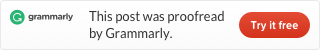# How to create a flexible calendar table with Power QueryHere is an example of how to generate calendar based on two input values – date of the first day and date of the last day.
It is a flexible approach you can generate a calendar for the year 2019 or only next 30 days.
M code that you can use in Blank Query is at the and of this post.The first task is to create two parameters for the beginning and end of the calendar table. Create a Blank Query, go to the Home -> Manage Parameters -> New Parameter and make start_date and end_date like in the picture below.Of course, you may have a different date format.

In Blank Query (by default Query1), you can generate a list of dates by using previously created parameters and duration calculation.
Just put this in the Formula Bar.
`= List.Dates(start_date, Duration.Days(end_date-start_date)+1, #duration(1, 0, 0, 0))`

You can also use a more flexible approach if you need to generate a list of fates using today’s date as a starting point.
`= List.Dates(DateTime.Date(DateTime.LocalNow()), Duration.Days(end_date-DateTime.Date(DateTime.LocalNow()))+1, #duration(1, 0, 0, 0))`

For new column calculations it is worth to mention:
– ISO weeknum from the previous post
– Text concatenation to add extra strings to the calculated value
`="Q " & Number.ToText([Quarter])`
– A combination of year and month
`=Date.Year([Date])*100+Date.Month([Date])`

You can also use Date.ToText function for flexibility because you can achieve many different results by using format code.
Month name
`=Date.ToText([Date],"MMMM")`
Week day name
`Date.ToText([Date],"dddd")`

For most of the calculations, I used Power Query date functions that you can see here.

You can also add some column with holiday marks by merging information from another table. If you don’t like to use an external source, then you can build that in another Blank Query.

After all your Workbook Queries pane should look like this.```//datacornering.com

let
Source = List.Dates(start_date, Duration.Days(end_date-start_date)+1, #duration(1, 0, 0, 0)),
#"Converted to Table" = Table.FromList(Source, Splitter.SplitByNothing(), null, null, ExtraValues.Error),
#"Renamed Columns" = Table.RenameColumns(#"Converted to Table",{{"Column1", "Date"}}),
#"Changed Type" = Table.TransformColumnTypes(#"Renamed Columns",{{"Date", type date}}),
#"Weekday Number" = Table.AddColumn(#"Changed Type", "Weekday Number", each Date.DayOfWeek([Date], Day.Monday)+1),
#"Weekday Name" = Table.AddColumn(#"Weekday Number", "Weekday Name", each Date.DayOfWeekName([Date], "en-US")),
#"ISO Week Number" = Table.AddColumn(#"Weekday Name", "ISO Week Number", each if
Number.RoundDown((Date.DayOfYear([Date])-(Date.DayOfWeek([Date], Day.Monday)+1)+10)/7)=0

then
Number.RoundDown((Date.DayOfYear(#date(Date.Year([Date])-1,12,31))-(Date.DayOfWeek(#date(Date.Year([Date])-1,12,31), Day.Monday)+1)+10)/7)

else if
(Number.RoundDown((Date.DayOfYear([Date])-(Date.DayOfWeek([Date], Day.Monday)+1)+10)/7)=53
and (Date.DayOfWeek(#date(Date.Year([Date]),12,31), Day.Monday)+1<4))

then
1

else
Number.RoundDown((Date.DayOfYear([Date])-(Date.DayOfWeek([Date], Day.Monday)+1)+10)/7)),
#"Month Number" = Table.AddColumn(#"ISO Week Number", "Month Number", each Date.Month([Date])),
#"Month Name" = Table.AddColumn(#"Month Number", "Month Name", each Date.MonthName([Date], "en-US")),
Quarter = Table.AddColumn(#"Month Name", "Quarter", each Date.QuarterOfYear([Date])),
#"Quarter Name" = Table.AddColumn(Quarter, "Quarter Name", each "Q " & Number.ToText([Quarter])),
Year = Table.AddColumn(#"Quarter Name", "Year", each Date.Year([Date])),
#"Year & Month" = Table.AddColumn(Year, "Year & Month", each Date.Year([Date])*100+Date.Month([Date]))
in
#"Year & Month"```
```//end_date
#date(2019, 12, 31) meta [IsParameterQuery=true, Type="Date", IsParameterQueryRequired=true]```
```//start_date
#date(2019, 1, 1) meta [IsParameterQuery=true, Type="Date", IsParameterQueryRequired=true]```